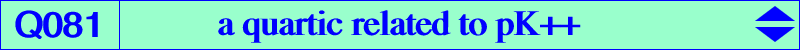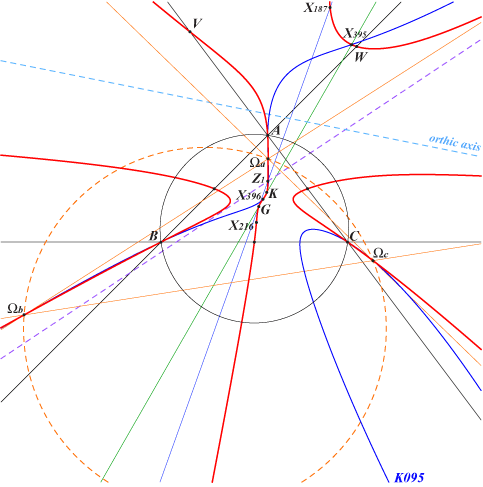too complicated to be written here. Click on the link to download a text file.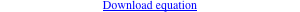X(2), X(6), X(187), X(216), X(395), X(396), X(8105), X(8106), X(14537) Z1 = X(14537) = X(2)X(187) /\ X(30)X(39) /\ X(32)X(381), etc midpoints of ABC U, V, W : their reflections in the corresponding feet of the orthic axis Ωa, Ωb, Ωc see Table 41 and CL052For any pole Ω different of K, there is a unique circular pivotal cubic pKc with pole Ω and for any Ω different of Ωa, Ωb, Ωc (see table 41 and CL052) there is a unique equilateral cubic pKe with pole Ω. These two cubics pKc and pKe generate a pencil F of pivotal cubics with pole Ω and pivot P on the line passing through the pivots Pc and Pe of pKc and pKe. In particular, this line contains G/Ω (ceva conjugate) and pK(Ω, G/Ω) is a pK+. This pencil contains a least one central cubic pK++ if and only if Ω lies on Q081 or on the Steiner inellipse. More precisely : – when Ω lies on the Steiner inellipse, one of the fixed points of the isoconjugation lies on the line at infinity and the pencil F contains a degenerate pK++ which is the union of the cevian lines of this infinite point. – when Ω lies on Q081, the line PcPe contains G and F contains a generally proper pK++. The pK+ has its pivot on the complement of Q050. The following table gives a selection of these cubics.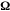pKc pKe pK++ pK+ line of pivots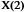K008 K092 medians of ABC X(2)X(187)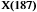K043 K042 pK(X187, X187÷X316) X(2)X(67)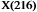pK(X216, X3153) K096 K044 pK(X216, X5) Euler line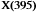K066b K046b pK(X395, X619) X(2)X(14)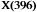K066a K046a pK(X396, X618) X(2)X(13)